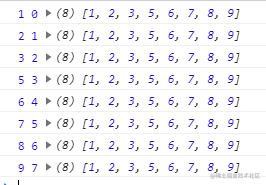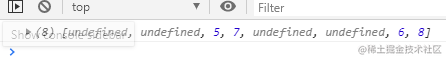# 一、Array 数组对象

## 1、push() 在数组尾部添加一个或者多个元素，并且返回数组的新长度

``````      arr=[1,2,3]
arr.push("a","b"); //插入字符串
arr.push(6); //在数组的尾部插入一个新元素
arr.push(6,7,8); //在数组的尾部插入多个新元素
a=arr.push(6,7,8); //通过添加到尾部元素后返回数组的新长度
console.log(arr);
console.log(a);

## 2、unshift() 在数组头部添加一个或者多个元素，并且返回数组的新长度

``````      arr=[1,2,3]
arr.unshift(0); //在数组的头部插入一个新元素
arr.unshift(-3,-2,-1,0); //在数组的头部插入多个新元素
var a=arr.unshift(-3,-2,-1,0); //返回数组的新长度
console.log(arr);
console.log(a);

## 3、pop() 删除数组尾部的最后一个元素，并且将这个被删除的元素返回

``````      arr=[1,2,3,4,5,6]
arr.pop(); //pop中没有参数,删除数组的最尾部一个元素
var a=arr.pop(); //pop删除数组 的最尾部一个元素，并且将被删除的元素返回
console.log(arr);
console.log(a);

## 4、shift() 删除数组的第一个元素，并且返回被删除的元素

``````      arr=[1,2,3,4,5,6]
arr.shift(); //将数组的第一个元素删除
var a=arr.shift(); //将数组的第一个元素删除，并且返回这个被删除的元素
console.log(arr);
console.log(a);

`不管删除还是添加都会改变数组的长度`

## 5、concat() 数组的合并，合并后会返回一个新数组，原来的两个数组不会变化

``````      var arr=[1,2,3,4];
var arr1=[5,6,7,8];
var arr2=arr.concat(arr1);  // 数组的合并，合并后会返回一个新数组，原来的两个数组不会变化
console.log(arr2);
var arr3=arr.concat(0,-1,-2); // 数组除了可以合并数组，也可以合并元素，将多个元素与原数组合并，返回新数组
console.log(arr3)
var arr4=arr.concat(0,arr1,["A","B"]); // concat既可以合并元素，也可以合并数组
console.log(arr4)
var arr5=arr.concat(); // 如果直接使用concat，就可以完成数组的复制功能
console.log(arr5)

## 6、join() 将数组的每个元素以指定的字符连接形成新字符串返回

``````      var arr=[1,2,3,4,5]; // join就是将数组的每个元素以指定的字符连接形成新字符串返回
var str=arr.join( ); // 将数组合并为字符串返回，默认使用逗号,连接
console.log(str);
var str=arr.join("|"); // 在这里join的参数是字符串的连接符v
console.log(str);
var str=arr.join(""); // ""作为连接符，会将数组元素首尾相连成为字符串
console.log(str);

## 7、splice() 这个方法可以从指定的位置删除给定数量的元素，并且在这个位置插入需要的元素，并且返回被删除的元素组成的新数组

splice(从什么位置开始,删除多少个元素,要插入的元素);

### 1）splice 刪除全部数组返回值

``````      var arr1=arr.splice(); // 没有任何参数时,返回一个空数组
console.log(arr1);
第一个参数是0，表示从第0位开始，第二个参数删除多少个没有填，意味着删除到尾部
var arr1=arr.splice(0); // 将所有数据转移到新数组
console.log(arr1);

### 2）splice 可以从前向后，也可以从后向前

``````      var arr1=arr.splice(0,3); // 从第0位开始删除3个元素，返回到新数组arr1
var arr1=arr.splice(-2); // 从第几位开始可以是负数，从后向前数（倒数）,因为没有给要删除的数量，因此删除到尾部
var arr1=arr.splice(0,1,-1); // 从数组的第0位开始，删除1个元素，并且在这个位置插入一个元素 -1,替换
var arr1=arr.splice(-1,1,0); // 数组的最后一位替换位0
var arr1=arr.splice(2,2,10,11); // 将数组中第二位开始两位元素替换为10，11

### 3）splice 可以插入一个，可以插入多个元素

``````      var arr=[1,2,3,4,5];
arr.splice(2,0,-1); // 在数组的第二位插入一个元素 -1
console.log(arr);
arr.splice(2,0,-1,-2,-3,-4); // 在数组的第二位插入多个元素
console.log(arr);

## 8、slice(start,end) 按指定位置截取复制数组的内容，返回新数组，不会改变原数组

``````      var arr1=arr.slice(1,4); // 从第1位开始截取到第4位之前
var arr1=arr.slice();   // 复制数组arr
var arr1=arr.slice(0);  // 复制数组
var arr1=arr.slice(3);  //从第三位截取到尾部复制
var arr1=arr.slice(-2); //从倒数第二位开始截取到尾部
var arr1=arr.slice(-3,-1); //从倒数第三位到倒数第一位

## 9、indexOf(要查询得元素,从什么位置开始查询) 位置就是下标

### 1）不能查询对象

``````      var arr=[{a:1},{a:2},{a:3},{a:4}];
var  index=arr.indexOf({a:1}); // 这是错误得，查找不到，因为查找得是新对象,地址不同
console.log(index);

### 2）查询所有相同的元素

``````      var arr=[1,3,1,2,3,5,2,3,4,6];
var index=arr.indexOf(3); // 这样只能查询到第一个3 （下标为1）
console.log(index);
var index=arr.indexOf(3,2); // 这样只能查询到第二个3 （下标为4）
console.log(index);

``````      var arr=[1,3,1,2,3,5,2,3,4,6];
var index=0; // 使用循环，查询到数组中所有为3的元素，并打印下标
while(true){
index=arr.indexOf(3,index);
console.log(index);
if(index===-1) break; // 查询到最后，查不到时返回-1  如果为-1 跳出循环，不再查询
index++;
}

## 10、Array.from(类似于数组的列表) 转为数组

### 1）根据标签名获取到标签列表 （获取到的是列表，不是数组，不能直接使用数组的方法

``````      var divs=document.getElementsByTagName("div"); // 获取html元素标签名是div的元素

divs.pop()；// 错误，不是数组不能直接使用数组的方法

var arr=Array.from(divs);  // ES6 的写法，把divs这个列表，转为数组
// ES5写法 =>  var arr=Array.prototype.slice.call(divs);

### 2）给所有 div 元素添加点击事件

``````      var divs=document.getElementsByTagName("div");
var arr=Array.from(divs); // 把获取到的所有div转成数组

for(var i=0;i<arr.length;i++){ // 遍历数组给每个div添加点击事件
arr[i].onclick=clickHandler;
}
function clickHandler(){
console.log(this);
var index=arr.indexOf(this);
console.log(index);  // 这样就可以判断点击得是列表中得第几个元素
}

## 11、lastIndexOf(查找得元素，从什么位置开始查找) 从后向前查找

``````      var arr=[1,3,1,2,3,5,2,3,4,6];
var index=arr.lastIndexOf(3);
console.log(index); // 打印下标 7

## 12、遍历数组（forEach 和 map）

### 1）forEach

`arr.forEach(function(数组中的元素，每个元素对应的下标，数组自身){ })`

``````      var arr=[1,2,3,5,6,7,8,9];
arr.forEach(function(a,b,c){
console.log(a,b,c);
})forEach没有返回值，使用return无效

### 2）map会返回一个与原数组长度相等的新数组

`arr.map(function(item,index,arr){ })`

``````      var arr=[3,5,7,9,1,2,4];
var arr2=arr.map(function(item,index,arr){
// console.log(item,index,arr);
// return "a";
return item+10;  // 在map中使用return 就是在对应的下标中添加对应的数据
});
console.log(arr2);

``````      // 遍历数组arr，将大于4的元素生成一个新的数组（新数组中会有undefined）
var arr=[1,3,5,7,2,4,6,8];
var arr1=arr.map(function(item){
if(item>4){
return item;
}
});
console.log(arr1);`map有返回值，与原数组等长的新数组，元素内容由return确定，不写return返回undefined`

## 13、arr.sort() 排序，仅能10以内数字

### 1）数值排序

``````      var arr=[1,3,5,7,2,4,6,8];
arr.sort(function(a,b){
return  a-b;  // 从小到大
// return  b-a;  // 从大到小
})
console.log(arr);

### 2）字符排序，先把字符转换成Unicode编码

``````      var arr=["n","c","g","h","a","j","y","k"];
arr.sort(function(a,b){   // 将字符排序
console.log(a.charCodeAt(0),b.charCodeAt(0)); // str.charCodeAt(0) => 将str字符串的第0项转换为Unicode编码
return a.charCodeAt(0)-b.charCodeAt(0);  // a-z
// return b.charCodeAt(0)-a.charCodeAt(0);  // z-a
})
console.log(arr);

## 14、some()

``````        var arr=[1,4,6,2,7,9,0];
var bool=arr.some(function(item,index,arr){  // 遍历数组，是否存在大于5的元素
return item>5;
});
console.log(bool);

## 15、every()

`var bool=arr.every(function(item,index,arr){ });`

``````        var arr=[1,4,6,2,7,9,0];
var bool=arr.every(function(item,index,arr){   // 判断数组中 是否所有的元素都大于2
return item>2;
});
console.log(bool);

## 16、filter()

``````        var arr1=arr.filter(function(item,index,arr){
return item>5;
});
console.log(arr1);

## 17、reduce()

``````        var arr=[10,3,4,7,3,5,8,9];
arr.reduce(function(value,item,index,arr){
// 循环的次数是数组的个数-1
// value就是上次遍历return的值，第0次遍历时（最开始时是数组的第0项）
console.log(value);
return value+1
})

reduce返回的是一个值，就是遍历到最后一次return出来的值

``````        var arr=[10,3,4,7,3,5,8,9];
var sum=arr.reduce(function(value,item){
return value+item;
});
console.log(sum);

``````        var arr=[10,3,4,7,3,5,8,9];
var sum=arr.reduce(function(value,item){
// 函数后面的参数就是累加的初始值
console.log(value);
return value+item;
},100);

## 18、Array.isArray()

``````        var arr=[1,2,3,4];
var obj={a:1};
console.log(typeof arr);
// 判断元素是否是数组，如果是数组返回true否则返回false，ES6
console.log(Array.isArray(arr));
console.log(Array.isArray(obj));

# 二、Math方法

## 1、π和根号2

``````      Math.PI; // Π
Math.SQRT2; // 根号2  常量，只能使用不能修改

## 2、取整

### 1）向下取整 Math.floor()

``````      a=Math.floor(25.6);
console.log(a);

### 2）向上取整 Math.ceil()

``````      b=Math.ceil(25.6);
console.log(b);

## 3、四舍五入 Math.round()

``````      c=Math.round(25.5);
console.log(c);

## 4、最大值和最小值

### 1）最小值Math.min()

``````      c=Math.min(4,7,8,3,1,9,6,0,3,2)
console.log(c)

2）最大值 Math.max()

``````      c=Math.max(4,7,8,3,1,9,6,0,3,2)
console.log(c)

## 5、随机数 Math.random

``````      c=Math.random()*10+1; // 随机1-10之间的任意数
console.log(c)

``````      c=Math.random()*10+1;
console.log(Math.floor(c)); // 向下取整

# 三、String 字符串方法

## 1、charAt()

``````      var str="abcdef";
console.log(str.charAt(3));

## 2、charCodeAt()

``````      var str="abcdef";
console.log(str.charCodeAt(2));

## 3、String.fromCharCode()

``````      n=String.fromCharCode(65); // 将Unicode编码转为字符串
console.log(n);

## 4、str.concat()

``````        var str="abc";
var str1="def";
var str2=str.concat(str1);   // var str2=str+str1; 这个等同于concat
console.log(str2);

## 5、 indexOf() 、lastIndexOf()

``````      var arr=[
{id:1001,name:"计算机",price:4999},
{id:1002,name:"电机",price:1999},
{id:1003,name:"记事本",price:9},
{id:1004,name:"课本",price:99},
{id:1005,name:"计算器",price:149},
];

// 模糊查找
var arr1=arr.filter(function(item){
return item.name.indexOf("本")>-1
});
console.log(arr1);

## 6、replace()

replace不修改原字符的内容，会返回一个被修改后的新字符串

``````      var str="abcdecf";
var str1=str.replace("c","z");
str.replace()
console.log(str,str1);

## 7、slice(start,end)

slice (从下标几开始，到下标几之前结束) 截取复制字符串

``````      var str="abcdecf";
var s=str.slice(1,2);
console.log(s);

## 8、substring()

``````      var str="abcdecf";
var s=str.substring(2,4); // 和slice相似
// substring不允许负数，所有的负值指0之前，因此负数都是0
// 可以逆向截取赋值
var s=str.substring(4,2);
console.log(s);

## 9、substr (从下标几开始，截取长度);

``````      var str="abcdecf";
var s=str.substr(-2,5); //从下标几开始，截取长度
console.log(s);

## 10、split(分隔符) 将字符串以分隔符进行分割转换为数组

``````      var str="a,b,c,d,e,f";
var arr=str.split(",");
console.log(arr);

## 11、reverse()；数组元素倒序或者反转，这是一个数组方法

``````      var str="abcdefg";
var str1=str.split("").reverse().join("");   //  连缀
console.log(str1)

## 12、字符串转换大小写

### 1）str.toLowerCase转为小写

``````      console.log("ABC".toLowerCase()); // 将字符串转换成小写

### 2）str.toUpperCase转为大写

``````      console.log("abc".toUpperCase()); // 将字符串转换成大写

# 三、日期对象

## 1、创建日期对象

``````      var date=new Date(); // 创建日期对象

## 2、获取日期

``````      var date=new Date();
date.getFullYear(); // 获取年份
date.getYear();   // 获取的年份是从1900年开始计算的，有问题
date.getMonth()+1; // 获取月份 从0开始
date.getDate();  // 获取日期
date.getDay();   // 获取星期  从0开始  0就是星期日 1是星期1
date.getHours();   // 获取小时
date.getMinutes(); // 获取分钟
date.getSeconds(); // 获取秒
date.getMilliseconds()；// 获取毫秒
console.log(date);

## 2、以上日期均可设置

``````      var date=new Date();
date.setFullYear(2021);
date.setMonth(12); // 1月，进位了年份+1 是从0开始的;
date.setDate(40); // 设置进位了
date.setHours(date.getHours() + 2); // 设置为现在时间的2小时后
date.getUTCFullYear(); // 凡是带有UTC都是格林尼治时间
date.toString(); // 直接转换为字符串
date.toLocaleString(); // 转换为本地（windows上）设置格式时间字符串
date.toUTCString(); // 转换为格林尼治时间字符串
console.log(date);

## 3、时间戳

``````      date.getTime(); // 毫秒数，是计算从1970年1月1日 00：00：00 到现在的毫秒数